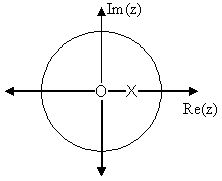Skip to main content

# 12.9: Discrete Time Filter Design

•• Contributed by Richard Baraniuk et al.
• Victor E. Cameron Professor (Electrical and Computer Engineering) at Rice University

## Estimating Frequency Response from Z-Plane

One of the primary motivating factors for utilizing the z-transform and analyzing the pole/zero plots is due to their relationship to the frequency response of a discrete-time system. Based on the position of the poles and zeros, one can quickly determine the frequency response. This is a result of the correspondence between the frequency response and the transfer function evaluated on the unit circle in the pole/zero plots. The frequency response, or DTFT, of the system is defined as:

\begin{align} H(w) &=\left.H(z)\right|_{z, z=e^{ j w}} \nonumber \\ &=\frac{\sum_{k=0}^{M} b_{k} e^{-(jwk)}}{\sum_{k=0}^{N} a_{k} e^{-(jw k)}} \end{align}

Next, by factoring the transfer function into poles and zeros and multiplying the numerator and denominator by $$e^{jw}$$ we arrive at the following equations:

$H(w)=\left|\frac{b_{0}}{a_{0}}\right| \frac{\prod_{k=1}^{M}\left|e^{j w}-c_{k}\right|}{\prod_{k=1}^{N}\left|e^{j w}-d_{k}\right|} \label{12.80}$

From Equation \ref{12.80} we have the frequency response in a form that can be used to interpret physical characteristics about the filter's frequency response. The numerator and denominator contain a product of terms of the form $$|e^{jw}-h|$$, where $$h$$ is either a zero, denoted by $$c_k$$ or a pole, denoted by $$d_k$$. Vectors are commonly used to represent the term and its parts on the complex plane. The pole or zero, $$h$$, is a vector from the origin to its location anywhere on the complex plane and $$e^{jw}$$ is a vector from the origin to its location on the unit circle. The vector connecting these two points, $$|e^{jw}-h|$$, connects the pole or zero location to a place on the unit circle dependent on the value of $$w$$. From this, we can begin to understand how the magnitude of the frequency response is a ratio of the distances to the poles and zero present in the z-plane as ww goes from zero to pi. These characteristics allow us to interpret $$|H(w)|$$ as follows:

$|H(w)|=\left|\frac{b_{0}}{a_{0}}\right| \frac{\prod \text { "distances from zeros" }}{\prod \text { "distances from poles" }}$

## Drawing Frequency Response from Pole/Zero Plot

Let us now look at several examples of determining the magnitude of the frequency response from the pole/zero plot of a z-transform. If you have forgotten or are unfamiliar with pole/zero plots, please refer back to the Pole/Zero Plots (Section 12.5) module.

Example $$\PageIndex{1}$$

In this first example we will take a look at the very simple z-transform shown below:

$H(z)=z+1=1+z^{-1}$

$H(w)=1+e^{-(j w)}$

For this example, some of the vectors represented by $$\left|e^{j w}-h\right|$$, for random values of $$w$$, are explicitly drawn onto the complex plane shown in Figure $$\PageIndex{1}$$ below. These vectors show how the amplitude of the frequency response changes as $$w$$ goes from $$0$$ to $$2 \pi$$, and also show the physical meaning of the terms in Equation \ref{12.80} above. One can see that when $$w=0$$, the vector is the longest and thus the frequency response will have its largest amplitude here. As ww approaches $$\pi$$, the length of the vectors decrease as does the amplitude of $$|H(w)|$$. Since there are no poles in the transform, there is only this one vector term rather than a ratio as seen in Equation \ref{12.80}.Figure $$\PageIndex{1}$$: The first figure represents the pole/zero plot with a few representative vectors graphed while the second shows the frequency response with a peak at $$+2$$ and graphed between plus and minus $$\pi$$.

Example $$\PageIndex{2}$$

For this example, a more complex transfer function is analyzed in order to represent the system's frequency response.

$H(z)=\frac{z}{z-\frac{1}{2}}=\frac{1}{1-\frac{1}{2} z^{-1}} \nonumber$

$H(w)=\frac{1}{1-\frac{1}{2} e^{-(j w)}} \nonumber$

Below we can see the two figures described by the above equations. The Figure $$\PageIndex{2}(a)$$ represents the basic pole/zero plot of the z-transform, $$H(w)$$. Figure $$\PageIndex{2}(b)$$ shows the magnitude of the frequency response. From the formulas and statements in the previous section, we can see that when $$w=0$$ the frequency will peak since it is at this value of ww that the pole is closest to the unit circle. The ratio from Equation \ref{12.80} helps us see the mathematics behind this conclusion and the relationship between the distances from the unit circle and the poles and zeros. As $$w$$ moves from $$0$$ to $$\pi$$, we see how the zero begins to mask the effects of the pole and thus force the frequency response closer to $$0$$.Figure $$\PageIndex{2}$$: The first figure represents the pole/zero plot while the second shows the frequency response with a peak at $$+2$$ and graphed between plus and minus $$\pi$$.

## Conclusion

In conclusion, using the distances from the unit circle to the poles and zeros, we can plot the frequency response of the system. As ww goes from $$0$$ to $$2\pi$$, the following two properties, taken from the above equations, specify how one should draw $$|H(w)|$$.

While moving around the unit circle...

1. if close to a zero, then the magnitude is small. If a zero is on the unit circle, then the frequency response is zero at that point.
2. if close to a pole, then the magnitude is large. If a pole is on the unit circle, then the frequency response goes to infinity at that point.